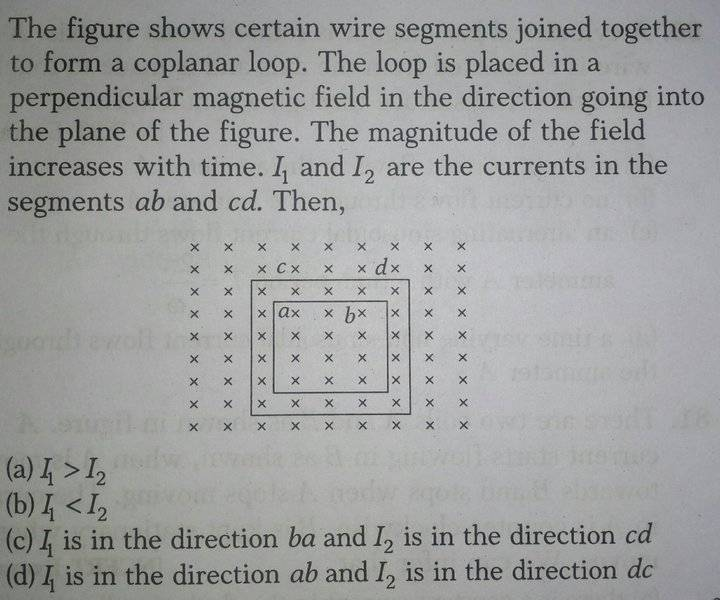# Magnetic field and coplanar loops

Jahnavi

## Homework Statement## The Attempt at a Solution

The current in the inner loop should flow in anticlockwise direction so as to resist the increase in flux of the external magnetic field .

But how do I deal with the outer loop since there is smaller loop inside it ?

I think current should flow in anticlockwise direction in the outer loop as well .

But none of the options have current flowing in same direction in both inner and outer loops . Also , how do I determine the magnitude of the two currents ?

#### Attachments

Homework Helper
Gold Member
The problem mentions "a coplanar loop", yet you mention an inner and an outer loop. Is there one rectangular loop of some finite width or two very thin wire loops? Assuming the latter, as you seem to be doing, is the magnetic flux through the outer loop going to be the same with and without the inner loop in place?

Jahnavi
Assuming the latter, as you seem to be doing, is the magnetic flux through the outer loop going to be the same with and without the inner loop in place?

I think , yes .

But this is exactly the confusion . What will be the area used while calculating induced EMF in the outer loop ? Is it the area of the outer loop as if the inner loop doesn't exist OR is it the area enclosed by the outer loop minus area enclosed by inner loop ?

Homework Helper
Gold Member
The current in the inner loop should flow in anticlockwise direction so as to resist the increase in flux of the external magnetic field .
But how do I deal with the outer loop since there is smaller loop inside it ?
Why should it make any difference? (We always assume the self-induced B field is << the externally applied field.)
I think current should flow in anticlockwise direction in the outer loop as well .
Good thought.
But none of the options have current flowing in same direction in both inner and outer loops.
3 of the 4 options are likely wrong, don't you think?
Also, how do I determine the magnitude of the two currents ?

•Jahnavi
Jahnavi

While applying Faraday in the outer loop , what area should I use , area of the outer loop OR area of the outer loop minus area of the inner loop ?

Homework Helper
Gold Member
The area of the outer loop is used to compite the EMF in the outer loop. The resulting currents in the loops are assumed to be small enough that their magnetic fields do not affect the magnetic field appreciably. Additional hint: The current is going to be equal to the EMF divided by the resistance, with the resistance proportional to the length of the loop of wire.

•Jahnavi and cnh1995
Homework Helper
Gold Member
While applying Faraday in the outer loop , what area should I use , area of the outer loop OR area of the outer loop minus area of the inner loop ?
Does the inner loop detract from the flux in the outer loop?

Homework Helper
Gold Member
This one needs one additional part to really determine it conclusively: Is ## \frac{xy}{2 (x+y)}>\frac{uv}{2(u+v)} ##, if ## x>u ## and ## y>v ##? (Basically dividing the area (EMF) by the perimeter (resistance). The ratio is proportional to the current). I believe I succeeded in doing an algebraic proof of this result.(##x,y,u,v ## are all >0). ## \\ ## @Jahnavi You might want to prove this yourself to show ## I_2>I_1 ##. ## \\ ## The proof involves one trick, and the rest follows very quickly: ## \\ ## In the inequality above, divide the numerator and denominator on the left side by ## xy ## , and divide the numerator and denominator on the right side by ## uv ## . (The 2's can be canceled to make things simpler). Then analyze the result algebraically.

Last edited:
•Jahnavi
Jahnavi
Thanks !

•Homework Helper
Gold Member
This one needs one additional part to really determine it conclusively: Is ## \frac{xy}{2 (x+y)}>\frac{uv}{2(u+v)} ##, if ## x>u ## and ## y>v ##? (Basically dividing the area (EMF) by the perimeter (resistance). The ratio is proportional to the current). I believe I succeeded in doing an algebraic proof of this result.(##x,y,u,v ## are all >0). ## \\ ## @Jahnavi You might want to prove this yourself to show ## I_2>I_1 ##. ## \\ ## The proof involves one trick, and the rest follows very quickly: ## \\ ## In the inequality above, divide the numerator and denominator on the left side by ## xy ## , and divide the numerator and denominator on the right side by ## uv ## . (The 2's can be canceled to make things simpler). Then analyze the result algebraically.
I assumed the loops were square in which case it is very easlily proved that I2 > I1. But, good point.

••